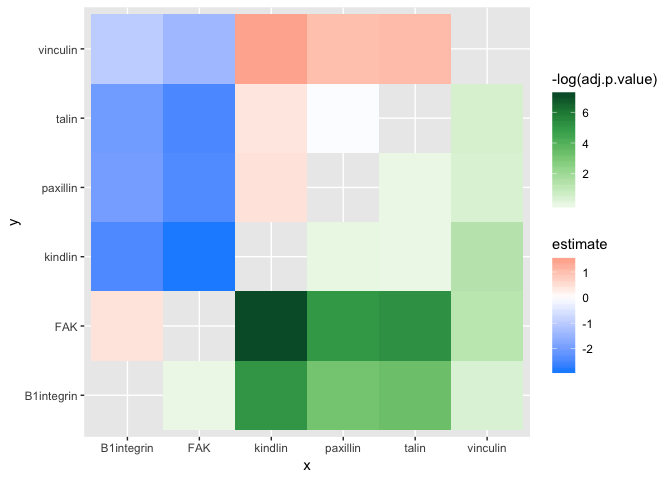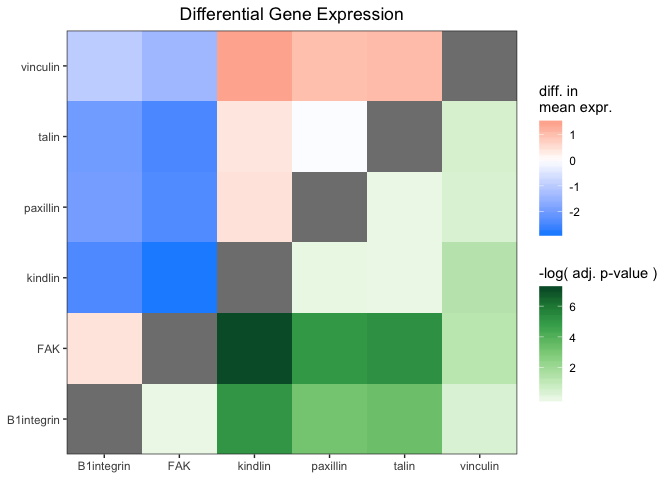# Statistical Test Plotting with ‘ggasym’

## Purpose

One of the great uses of ‘ggasym’ is to plot two values from the results of a multi-way statistical test. Each comparison is a cell, and two values can be used for the fills. Below I give brief examples and plot the differences in mean and the p-value on the symmetric matrix.

library(dplyr)
library(ggplot2)
library(tibble)
library(purrr)
library(broom)
library(ggasym)

## Data

The data will be modeled as expression values of 6 genes, each with 10 measurements. I will then as if any of them have different levels of expression.

n_reps <- 10  # number of measurements per gene
expt_std_dev <- 1.5  # std. dev. of measurements
genes <- c("FAK", "talin", "paxillin", "vinculin", "B1integrin", "kindlin")
# "real" expression levels to be used as the mean in rnorm
real_expression_levels <- sample(seq(1, 5, 0.1), length(genes), replace = TRUE)
# create a tibble
expr_data <- tibble(gene = rep(genes, n_reps),
real_expr = rep(real_expression_levels, n_reps),
rep_num = sort(rep(1:n_reps, length(genes))))
# add in the measured expression values as a normal distribution around the mean
expr_data <- expr_data %>%
mutate(expt_expr = rnorm(nrow(expr_data),
mean = real_expr,
sd = expt_std_dev))
#> # A tibble: 6 x 4
#>   gene       real_expr rep_num expt_expr
#>   <chr>          <dbl>   <int>     <dbl>
#> 1 FAK              4.6       1     6.51
#> 2 talin            2         1     2.62
#> 3 paxillin         2.5       1     0.190
#> 4 vinculin         3.3       1     1.91
#> 5 B1integrin       4.7       1     4.26
#> 6 kindlin          1.8       1     1.79

## Statistical Tests

I then use an ANOVA to test if there are any differences between any of the comparisons of gene expression.

res_aov <- aov(expt_expr ~ gene, data = expr_data)
broom::tidy(res_aov)
#> # A tibble: 2 x 6
#>   term         df sumsq meansq statistic    p.value
#>   <chr>     <dbl> <dbl>  <dbl>     <dbl>      <dbl>
#> 1 gene          5  68.7  13.7       6.45  0.0000911
#> 2 Residuals    54 115.    2.13     NA    NA

With a very low p-value from the ANOVA, I run the Tukey post-hoc test to find which genes are at different levels.

tukey_res <- TukeyHSD(res_aov)
tukey_res
#>   Tukey multiple comparisons of means
#>     95% family-wise confidence level
#>
#> Fit: aov(formula = expt_expr ~ gene, data = expr_data)
#>
#> \$gene
#>                            diff        lwr         upr     p adj
#> FAK-B1integrin       0.42786034 -1.5000734  2.35579405 0.9859350
#> kindlin-B1integrin  -2.41258042 -4.3405141 -0.48464671 0.0064520
#> paxillin-B1integrin -1.96884001 -3.8967737 -0.04090630 0.0426958
#> talin-B1integrin    -2.02075178 -3.9486855 -0.09281807 0.0347905
#> vinculin-B1integrin -0.97209337 -2.9000271  0.95584034 0.6721614
#> kindlin-FAK         -2.84044076 -4.7683745 -0.91250705 0.0008182
#> paxillin-FAK        -2.39670035 -4.3246341 -0.46876664 0.0069377
#> talin-FAK           -2.44861212 -4.3765458 -0.52067841 0.0054659
#> vinculin-FAK        -1.39995371 -3.3278874  0.52798000 0.2803309
#> paxillin-kindlin     0.44374040 -1.4841933  2.37167411 0.9834395
#> talin-kindlin        0.39182864 -1.5361051  2.31976234 0.9905661
#> vinculin-kindlin     1.44048704 -0.4874467  3.36842075 0.2513411
#> talin-paxillin      -0.05191177 -1.9798455  1.87602194 0.9999995
#> vinculin-paxillin    0.99674664 -0.9311871  2.92468035 0.6484209
#> vinculin-talin       1.04865841 -0.8792753  2.97659211 0.5976093

## Plotting

Now I want to plot the estimate in the top-left and p adj in the bottom right. First, I must prepare the data for use with geom_asymmat() by passing the results of the Tukey post-hoc test to asymmetrise_stats(). You can see that it returns the data in a tibble with new columns x and y that are the result of splitting comparison.

asymmat_tib <- asymmetrise_stats(tukey_res)
#> # A tibble: 6 x 8
#>   term  comparison    estimate conf.low conf.high adj.p.value x      y
#>   <chr> <chr>            <dbl>    <dbl>     <dbl>       <dbl> <chr>  <chr>
#> 1 gene  FAK-B1integr…    0.428    -1.50    2.36      0.986    FAK    B1int…
#> 2 gene  kindlin-B1in…   -2.41     -4.34   -0.485     0.00645  kindl… B1int…
#> 3 gene  paxillin-B1i…   -1.97     -3.90   -0.0409    0.0427   paxil… B1int…
#> 4 gene  talin-B1inte…   -2.02     -3.95   -0.0928    0.0348   talin  B1int…
#> 5 gene  vinculin-B1i…   -0.972    -2.90    0.956     0.672    vincu… B1int…
#> 6 gene  kindlin-FAK     -2.84     -4.77   -0.913     0.000818 kindl… FAK

Finally, I can plot the data using geom_asymmat().

ggplot(asymmat_tib, aes(x = x, y = y)) +
geom_asymmat(aes(fill_tl = estimate, fill_br = -log(adj.p.value))) +
scale_fill_tl_gradient2(low = "dodgerblue", high = "tomato") +
scale_fill_br_distiller(type = "seq", palette = "Greens", direction = 1)And add a few styling changes with normal ‘ggplot2’ semantics.

ggplot(asymmat_tib, aes(x = x, y = y)) +
geom_asymmat(aes(fill_tl = estimate, fill_br = -log(adj.p.value))) +
scale_fill_tl_gradient2(low = "dodgerblue", high = "tomato",
guide = guide_colourbar(order = 1)) +
scale_fill_br_distiller(type = "seq", palette = "Greens", direction = 1,
guide = guide_colourbar(order = 2)) +
theme_bw() +
theme(panel.background = element_rect(fill = "grey50"),
panel.grid = element_blank(),
axis.title = element_blank(),
plot.title = element_text(hjust = 0.5)) +
labs(title = "Differential Gene Expression",
fill_tl = "diff. in\nmean expr.",
fill_br = "-log( adj. p-value )") +
scale_x_discrete(expand = c(0,0)) +
scale_y_discrete(expand = c(0,0))One of the conclusions that can be drawn here is that the difference in expression of kindlin and FAK is the greatest and has a very low adjusted p-value. Thus, one of the conclusion is that kindlin is expressed at a lower level than FAK.